output.to from Sideway
Draft for Information Only

# Content

`Python Numeric Literal Integer Literal  Definition of Integer Literal  Features of Integer  Python Integer Catalogue Floating Point Literal  Definition of Floating Point Literal  Features of Floating Point  Python Floating Point Catalogue Imaginary Literal  Definition of Imaginary Literal  Features of Imaginary Source and Reference`

# Python Numeric Literal

Python supports numeric literals of integers, floating point numbers, and imaginary numbers. Complex literal is formed by adding a real number and an imaginary number. Besides, numeric sign is not included in numeric literal, but numeric sign '-' or '+' is considered as an unary operator - or +.

## Integer Literal

An integer literal is a sequence of ASCII characters.

### Definition of Integer Literal

The definition of integer literal is: integer::=decinteger | bininteger | octinteger | hexinteger decinteger::=nonzerodigit (["_"] digit)* | "0"+ (["_"] "0")* bininteger::="0" ("b" | "B") (["_"] bindigit)+ octinteger::="0" ("o" | "O") (["_"] octdigit)+ hexinteger::="0" ("x" | "X") (["_"] hexdigit)+ nonzerodigit::="1"..."9" digit::="0"..."9" bindigit::="0" | "1" octdigit::="0"..."7" hexdigit::=digit | "a"..."f" | "A"..."F"

### Features of Integer

Leading zeros in a non-zero decimal number are not allowed. The length of integer literal is unlimited and is only limited by available memory. Therefore, long integer type is no longer necessary. Underscores _ are only used to group digits for enhancing readability and underscores are ignored for determining the numeric value of the literal. One underscore can occure between digits, but must be placed after base specifiers like 0x.

### Python Integer Catalogue

Python integer can be divided into decinteger, bininteger, octinteger, and hexinteger. decintegerLeading zeros in a non-zero decimal literal are not allowed to prevent disambiguation with C-style octal literals. Underscore _ can be placed in between any two decimal characters of an integer literal. Decimal characters can be any nonzerodigit, "1"..."9" and digit, "0"..."9".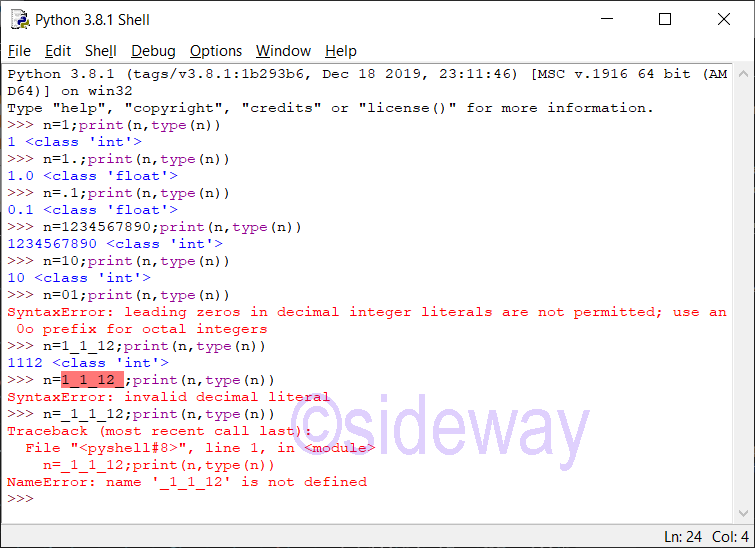binintegerThe base specifiers for binary integer are 0b and 0B. Underscore _ can be placed in between any two binary characters of an bininteger literal after the base specifier. Binary characters after base specifier can be any bindigit, "0" | "1" after the base specifier.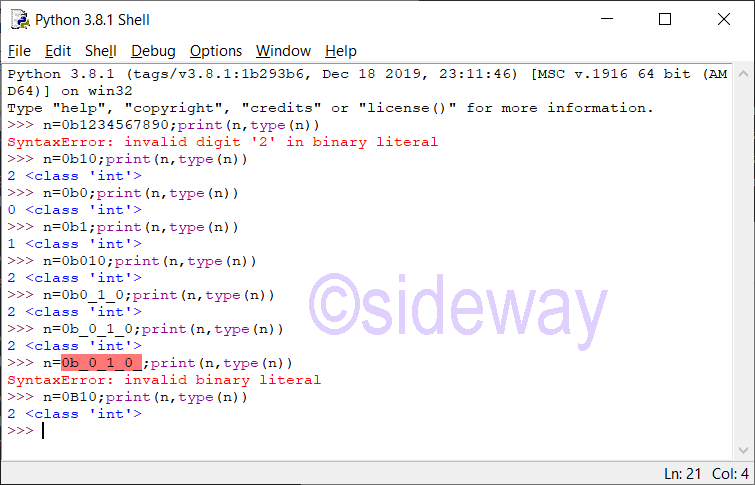octintegerThe base specifiers for octal integer are 0o and 0O. Underscore _ can be placed in between any two octal characters of an octinteger literal after the base specifier. Octal characters after base specifier can be any octinteger, "0"..."7"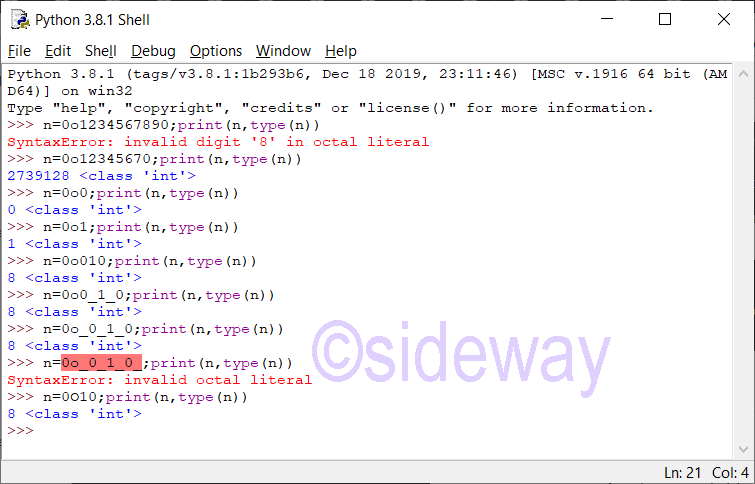hexintegerThe base specifiers for hexadecimal integer are 0x and 0X. Underscore _ can be placed in between any two hexadecimal characters of an hexinteger literal after the base specifier. Hexadecimal characters after base specifier can be any hexdigit, "0"..."9" | "a"..."f" | "A"..."F"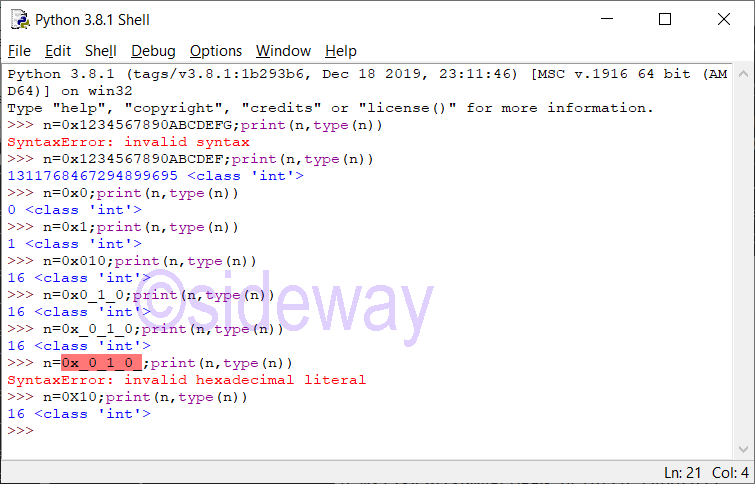## Floating Point Literal

A floating point literal is a sequence of characters for representing an floating point number with an integer part, a fractional part, and an exponent part.

### Definition of Floating Point Literal

The definition of floating point literal is: floatnumber::=pointfloat | exponentfloat pointfloat::=[digitpart] fraction | digitpart "." exponentfloat::=(digitpart | pointfloat) exponent digitpart::=digit (["_"] digit)* fraction::="." digitpart exponent::=("e" | "E") ["+" | "-"] digitpart

### Features of Floating Point

Leading zeros in a floating point literal are allowed because the integer and exponent parts are always interpreted using radix 10. The allowed range of floating point literals is implementation-dependent. Underscores _ are only used to group digits for enhancing readability and underscores are ignored for determining the numeric value of the literal. One underscore can occure between numeric digits.

### Python Floating Point Catalogue

pointfloatDecimal point . is used together with digits to present the floating point literal. A pointfloat is always composed of a digitpart and a fraction. A fraction is specified by a decimal point .. Therefore decimal point . can be placed at the end of floating point literal to indicate a floating point literal. Leading zeros in a floating point literal are allowed. Underscores _ are only used to group digits for enhancing readability and underscores are ignored for determining the numeric value of the literal. One underscore can occure between numeric digits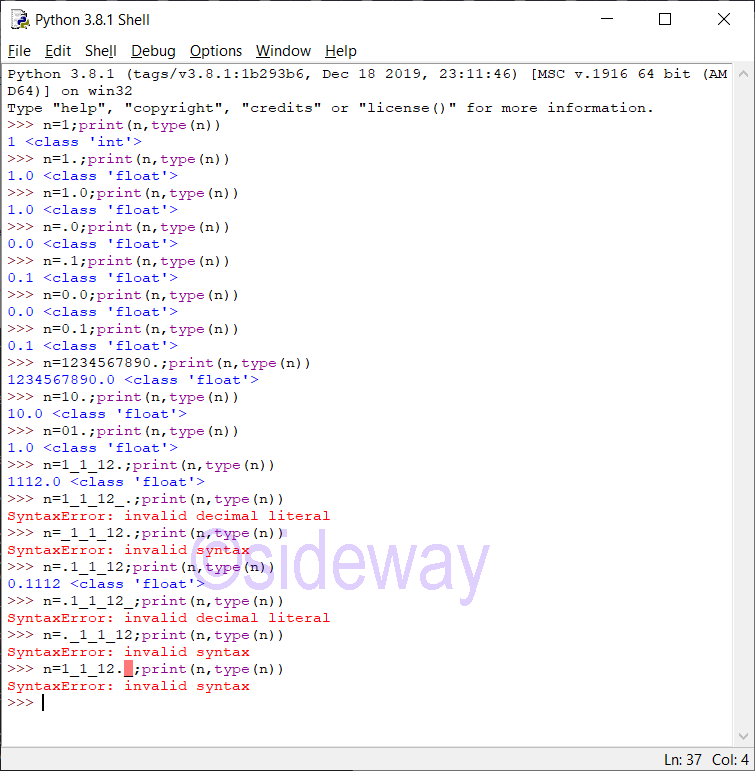exponentfloatExponent e or E is used together with digits to present the floating point literal. For a exponentfloat, the exponent part must be included in the floating point literal, while digit part of a floating point literal can be a pointfloat or simple digitpart. A exponent is specified by a character e or E followed only by a digitpart and a sign operator + or - can be added before the digitpart.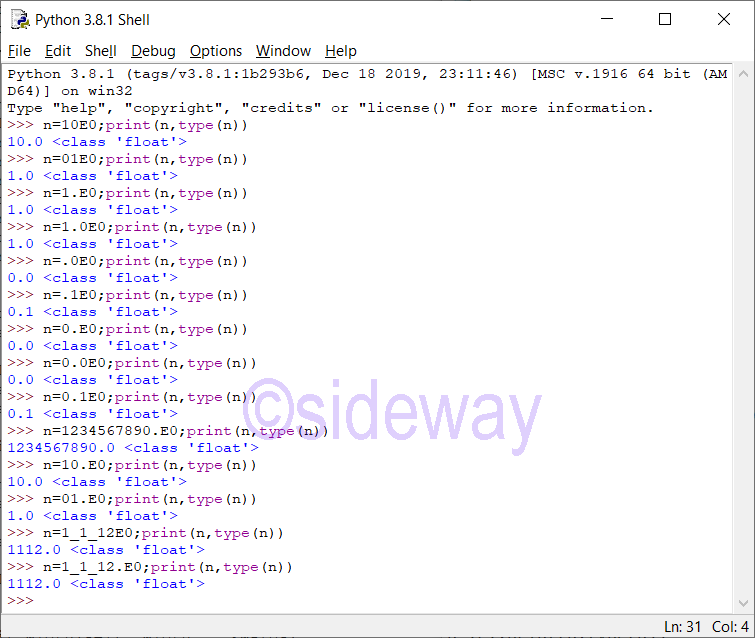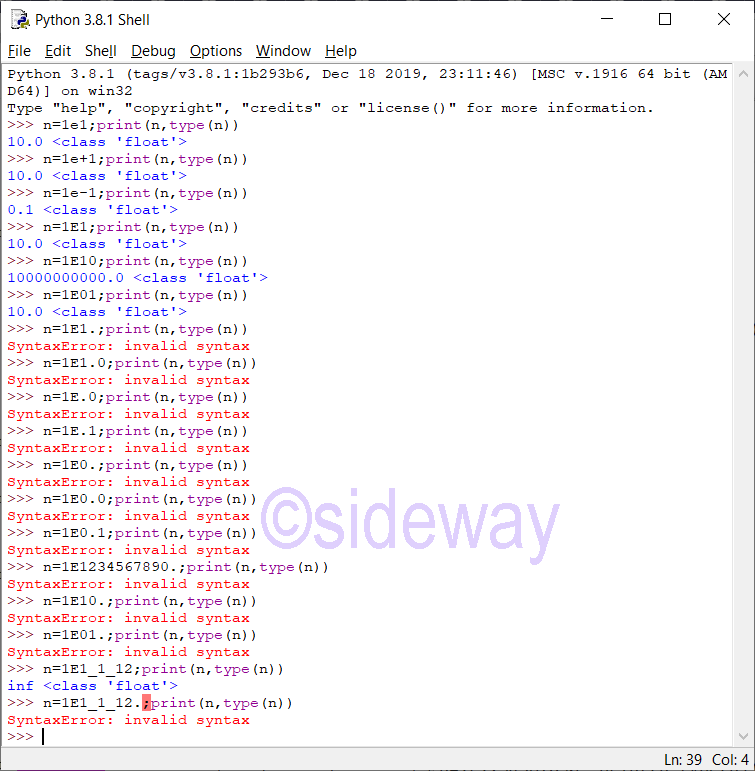## Imaginary Literal

An imaginary literal is a sequence of characters for representing an imaginary number of the imaginary part of a complex number.

### Definition of Imaginary Literal

The definition of imaginary literal is: imagnumber::=(floatnumber | digitpart) ("j" | "J")

### Features of Imaginary

An imagnumber is actually a floatnumber or digitpart with a imaginary specifier j or J. In other words, an imagnumber have the same proerties and restrictions as a floatnumber or digitpart. An imaginary literal can be used to construct a complex number by adding a floating point number to it.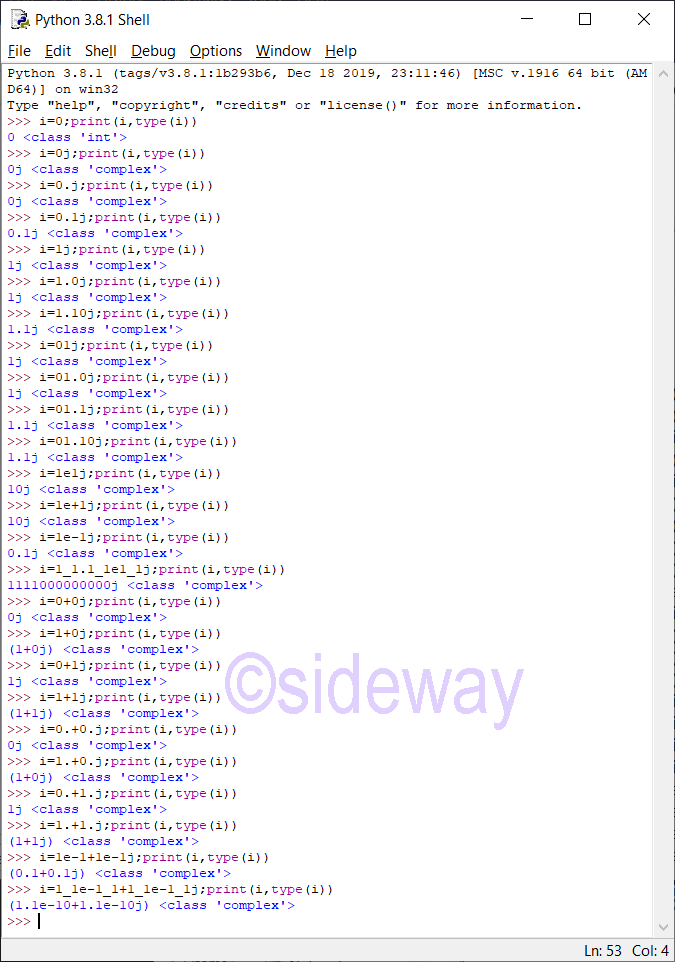## Source and Reference

ID: 210100004 Last Updated: 4/1/2021 Revision: 0Home 5

Management

HBR 3

Information

Recreation

Culture

Chinese 1097

English 337

Computer

Hardware 151

Software

Application 202

Latex 10

Manim 159

Numeric 19

Programming

Web 285

Unicode 495

HTML 65

CSS 59

ASP.NET 194

OS 391

Python 46

Knowledge

Mathematics

Algebra 29

Geometry 18

Calculus 67

Engineering

Mechanical

Rigid Bodies

Statics 92

Dynamics 37

Control

Natural Sciences

Electric 27